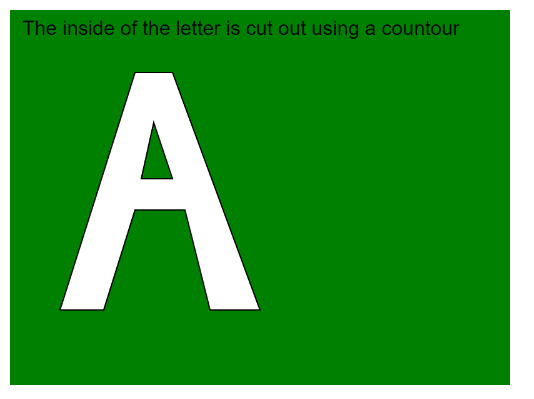# p5.js | endContour() Function

• Last Updated : 27 May, 2022

The endContour() function in p5.js is used to stop the recording of vertices when creating a contour using the beginContour() function. These functions are used to remove a portion of a shape from another shape. The vertices of the interior shape have to be defined in the opposite direction to that of the exterior one. If the exterior shape has the vertices defined in the clockwise order, then the interior shape has to be defined in the counter-clockwise direction. The recording of the interior shape is then stopped by using this function. This function can only be used inside the beginShape() or endShape() function. Transformations like translate(), rotate() and scale() do not work with shapes and contours.

Syntax:

`endContour()`

Parameters: This function accepts no parameters.

The program below illustrates the endContour() function in p5.js:

Example:

## javascript

 `function` `setup() {``  ``createCanvas(400, 300);``  ``textSize(16);``}` `function` `draw() {``  ``clear();``  ``background("green");``  ``text("The inside of the letter is cut out"+``       ``" using a contour", 10, 20);` `  ``// Starting the shape``  ``// using beginShape()``  ``beginShape();` `  ``// Specifying all the vertices``  ``// of the exterior shape``  ``vertex(40, 240);``  ``vertex(100, 50);``  ``vertex(130, 50);``  ``vertex(200, 240);``  ``vertex(160, 240);``  ``vertex(140, 160);``  ``vertex(100, 160);``  ``vertex(75, 240);` `  ``// Starting a contour``  ``beginContour();` `  ``// Specifying all the vertices``  ``// of the interior shape``  ``// in counter-clockwise order``  ``vertex(130, 135);``  ``vertex(115, 90);``  ``vertex(105, 135);` `  ``// Ending the contour``  ``// using endContour()``  ``endContour();` `  ``// Ending the shape``  ``endShape(CLOSE);``}`

Output:Online editor: https://editor.p5js.org/

My Personal Notes arrow_drop_up